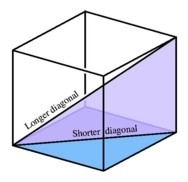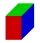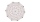# Wall and body diagonals

The block/cuboid has dimensions a = 4cm, b = 3cm and c = 12cm. Calculates the length of the wall and body diagonals.

u1 =  5 cm
u2 =  12.3693 cm
u3 =  12.6491 cm
d =  13 cm

### Step-by-step explanation:Did you find an error or inaccuracy? Feel free to write us. Thank you!Tips to related online calculators
Pythagorean theorem is the base for the right triangle calculator.

#### You need to know the following knowledge to solve this word math problem:

We encourage you to watch this tutorial video on this math problem:

## Related math problems and questions:

• Body diagonalCalculate the length of the body diagonal of a block with dimensions: a = 20 cm, b = 30 cm, c = 15 cm.
• Body diagonalCalculate the volume of a cuboid whose body diagonal u is equal to 6.1 cm. Rectangular base has dimensions of 3.2 cm and 2.4 cm
• Cuboid face diagonalsThe lengths of the cuboid edges are in the ratio 1: 2: 3. Will the lengths of its diagonals be the same ratio? The cuboid has dimensions of 5 cm, 10 cm, and 15 cm. Calculate the size of the wall diagonals of this cuboid.
• Cuboid easyThe cuboid has the dimensions a = 12 cm, b = 9 cm, c = 36 cm. Calculate the length of the body diagonal of the cuboid.
• Body diagonalFind the length of the body diagonal of a cuboid with edges lengths of 16 cm, 7 cm, and 4 cm.
• Concrete blockDetermine the volume of the concrete block whose one edge of the base has a length of 3 meters, body diagonal is 13 meters, and its height is 12 meters.
• Height of the cuboidCuboid with a rectangular base, measuring 3 cm and 4 cm diagonal has a body 13 centimeters long. What is the height of the cuboid?
• Diagonals of a prismThe base of the square prism is a rectangle with dimensions of 3 dm and 4 dm. The height of the prism is 1 m. Find out the angle between the body diagonal with the diagonal of the base.
• Cuboid - volume, diagonalsThe length of the one base edge of cuboid a is 3 cm. Body diagonal is ut=13 cm and diagonal of cuboid's baseis u1=5 cm. What is the volume of the cuboid?
• Prism 4 sidesThe prism has a square base with a side length of 3 cm. The diagonal of the sidewall of the prism/BG/is 5 cm. Calculate the surface of this prism in cm square and the volume in liters
• Cuboid diagonalsThe cuboid has dimensions of 15, 20 and 40 cm. Calculate its volume and surface, the length of the body diagonal and the lengths of all three wall diagonals.
• Body diagonalCuboid with base 7cm x 3,9cm and body diagonal 9cm long. Find the height of the cuboid and the length of the diagonal of the base,
• Wall and body diagonalsCalculate the lengths of the wall and body diagonals of the cuboid with edge dimensions of 0.5 m, 1 m, and 2 m
• Space diagonal anglesCalculate the angle between the body diagonal and the side edge c of the block with dimensions: a = 28cm, b = 45cm and c = 73cm. Then, find the angle between the body diagonal and the plane of the base ABCD.
• Cube diagonalsThe cube has a wall area of 81 cm square. Calculate the length of its edge, wall, and body diagonal.
• Ratio of edgesThe dimensions of the cuboid are in a ratio 3: 1: 2. The body diagonal has a length of 28 cm. Find the volume of a cuboid.
• Distance of linesFind the distance of lines AE, CG in cuboid ABCDEFGH, if given | AB | = 3cm, | AD | = 2 cm, | AE | = 4cm